Lorem ipsum dolor sit amet, consectetur adipiscing elit, sed do eiusmod tempor incididunt ut labore.

15 St Margarets, NY 10033
(+381) 11 123 4567
ouroffice@aware.com

### KMF#### Binary Search in Python – DZone Web Dev

Binary search is the search technique that helps to make the searching faster. So for understanding the binary search method implementation in Python, let’s first understand linear search?

In this article, we will answer the following questions: What the binary search is? How is it derived? How does it search faster?

## What Is Linear Search?

Linear search is the simple searching technique in which we search for the data in the given Array or Linked List. T^his searches elements sequentially by comparing the element one at a time. And perform the comparison till the last element of an array.

### Algorithm for Linear Search

1. Loop i = 1 to end of Array:
2. Compare the key value with the value at the array of ith index
3. If the value match then returns the index.

For example – Given an array of elements – A = [5, 8, 9, 2, 4, 1, 3, 7]. And a key = 4. Now we need to search whether the key is present in the given array or not.

### Explanation of the Algorithm

So for this problem, How linear search works as follows:

At the first step, it compares the key value with the array index. Not matching then go to the next step.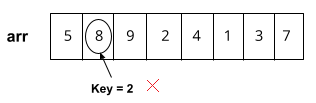The second step follows the same step as the first one. Not matching, continue the step.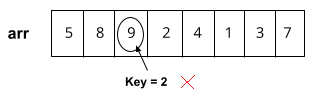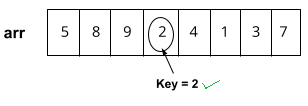In the fourth step, the value was found, so we will return the index of the array where the element is found.

### Python Implementation: Run Code

``````def linearsearch(arr, key):
#Loop through the array
for i in range(len(arr)):
#comparing value at each index
if arr[i] == key:
#if value found then return the index
return i
return -1

if __name__ == "__main__":
arr = [5, 8, 9, 2, 4, 1, 3, 7]
key = 4
index = linearsearch(arr, key)
if index != -1:
print("Element found at index = ",index)
else:

``````Input 1 -  arr = [5, 8, 9, 2, 4, 1, 3, 7]
key = 4
Output 1 - Element found at Index 4

Input 2 -  arr = [8, 2, 7, 9, 4, 1, 6, 5]
key = 5
Output 2 - Element found at Index 7

Input 3 -  arr = [3, 2, 8, 9, 7, 6, 4, 1]
key = 5

### Time Complexity Analysis of Linear Search

• We are traversing the whole array at once for comparing the value. Ao if the key is found at the first index itself then it will be the best case. And loop will run only one step. So the time complexity for the Best Case is O( 1 ).
• Now consider a scenario if the key doesn’t exist in the array or is found at the last index then in the case the whole array needs to be traversed. That is the worst case. So time complexity for the Worst case is O ( n ).
• Now for the element found in the middle of the array or somewhere between, but not last. Then that will be the average case. And we don’t know where the key exists. Then the Average case is O ( n ).

### Space Complexity Analysis of Linear Search

In the algorithm, we are not taking any extra space for comparing the value except the 1 constant index pointer. So the Space complexity is O ( 1 ).

Now we have seen the time complexity of the linear search that is linear-time taking algorithm. So can we somehow reduce the time complexity of the linear-search technique?

Yes, that is what the binary search algorithm helps to achieve.

## What Is Binary Search?

Binary search is the searching technique that helps to search the key in the array. There is the only condition with the binary search algorithm, and that is the array element must be sorted. The binary search uses the technique of half interval search. And binary search algorithm can be applied in two different approaches –

1. Recursive Binary Search.
2. Iterative Binary Search.

Recursive Binary search algorithms use recursion to search and Iterative algorithms use a loop for searching.

### The Intuition of Binary Search

Consider an array of elements that are already in sorted order.  arr = [2, 3, 8, 9, 10, 15]. And Key = 10.  So the general algorithm for binary search is –

### Algorithm for Binary Search

• Pointers low = starting index,  high = last index,
• Find the mid index. And check if the key is found at mid index.
• If the key doesn’t exist in the mid then compare the key if it is less than or greater than the element at mid index.
• If the key is less than the mid index value then update the high to mid – 1 index and continue from step 2.
• If the key is greater than the mid-index value then update the low to mid + 1 index and continue from step 2.
• If found that low index is greater than the high index then return -1 indicating element is not present in the array.

### Explanation

So for this example, how binary search works as: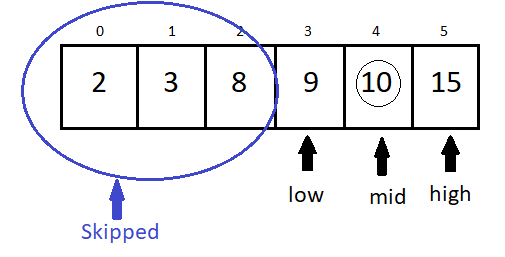• At the first step, we calculated mid = (low+high)/2. And compares the value if it matches with key = 10. It is not matching. Then we check that key is less than or greater than the mid index value. And we found that (10 > 8), so update low = mid + 1.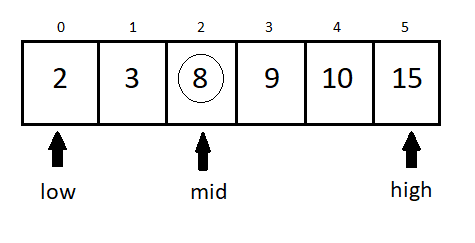• Now low is at index 3 and again calculated mid = (low + high) / 2. And we found that key exists at the index if mid so we will return that index.

## How Is Binary Search Faster?

And here in the binary search algorithm, using the knowledge that the element is already sorted, we have skipped checking the element from index zero to mid.

As we know that the key is greater than the mid-index value, then there is no need to check the elements between these indexes. And that’s what the binary search algorithm does.

It skips the unnecessary comparisons on the array and saves the computation time of the CPU. That makes this binary search algorithm faster.

### 1. Recursive Python Code for Binary Search: Run Code

``````def binarySearchRecursive(arr, low, high, key):
#Base case to terminate recursion
if(low > high):
return -1
#Calculating the mid index
mid = (low + high)//2

#Comparing that if element found at index mid
if(arr[mid] == key):
#Element found the return mid index
return mid

#Comparing if key is less than mid index
elif(key < arr[mid]):
#Recursively calling the search on the left subarray from  mid
return binarySearchRecursive(arr, low, mid-1, key)
else:
#Recursively calling the search on the right subarray from  mid
return binarySearchRecursive(arr, mid+1, high, key)

if __name__ == "__main__":
arr = [2, 4, 6, 8, 9, 10, 12]
key = 6
index = binarySearchRecursive(arr, 0, len(arr)-1, key)
if index != -1:
print("Element found at index = ",index)
else:

``````Input 1 - arr = [2, 4, 6, 7, 8, 9]
Key = 7
Output -  Element found at index 3

Input 2 - arr = [2, 4, 6, 8, 9, 10, 12]
key = 6
Output -  Element found at index 2

Input 2 - arr = [5, 6, 9, 17, 19, 21]
key = 10

### 2. Iterative Binary Search Python Implementation: Run Code

``````def iterativeBinarySearch(arr, key):
#Declaring variable to point on the array
low = 0
high = len(arr)-1

#loop until the search indexes are valid
while low <= high:
#calculating mid index
mid = (low + high)//2

#comparing if element found at mid index
if(arr[mid] == key):
#element found then return the mid index
return mid

#Comparing if the key is less than or greater than the mid index value
elif(key < arr[mid]):
#Updating high to mid - 1 and searching on the left sub array.
high = mid - 1

else:
#Updating low to mid+1 and searching on the right subarray.
low = mid + 1

#Returning -1 index indicating element fow found in the array
return -1;

if __name__ == "__main__":
arr = [2,4,6,8,10,12]
key = 12
index = iterativeBinarySearch(arr, key)
if index != -1:
print("Element found at index = ", index)
else:

``````Input 1 - arr = [2, 4, 6, 7, 8, 9]
Key = 7
Output -  Element found at index 3

Input 2 - arr = [2, 4, 6, 8, 9, 10, 12]
key = 6
Output -  Element found at index 2

Input 2 - arr = [5, 6, 9, 17, 19, 21]
key = 10

### Time Complexity Analysis of Binary Search

• We are calculating the mid-index of the array and comparing the mid-index. If a key exists at the mid index, then we return the index. So consider a scenario when the key we are searching is present at the initial mid-index, then we return the mid index. And that will be the best case because no more comparison will be done. So we can conclude that the Best Case is – O ( 1 ).
• The functionality of the binary search algorithm is that, in every iteration, we are skipping the half array and checking for the key. First, it checks for the middle element, and then according to key comparison, we are skipping half of the array. The steps are –
1. [2, 4, 6, 8, 10, 12]     Key = 12
2. [8, 10, 12]     Key = 12
3. [10, 12]    key = 12
4.     Element Found

So in the above steps on each comparison, the array is divided in half. So the time complexity for both the Average Case and Worst case is – O ( log2 n).

Note: The Time complexity analysis for both recursive and Iterative versions will be the same.

### Space Complexity Analysis of Binary Search

• We are not taking any extra space for searching an element in an array except for some constant pointers. So the Space Complexity of the binary search is O( 1 ).
• If we talk about the recursive algorithm of binary search then it also does not take any additional space except some constant pointers. But we know that recursion uses an internal stack (call stack) for executing the function. And the recursive function is called utmost O( log2 n) times by analyzing the time complexity. So the space complexity of the recursive binary search algorithm is O ( log2 n).

### How Efficient Is Binary Search as Compared to Linear Search?

The binary search algorithm takes O(log n) times to search and the linear search take O(n) times to search an element. So for understanding this asymptotic complexity of these two algorithms, consider the below graph.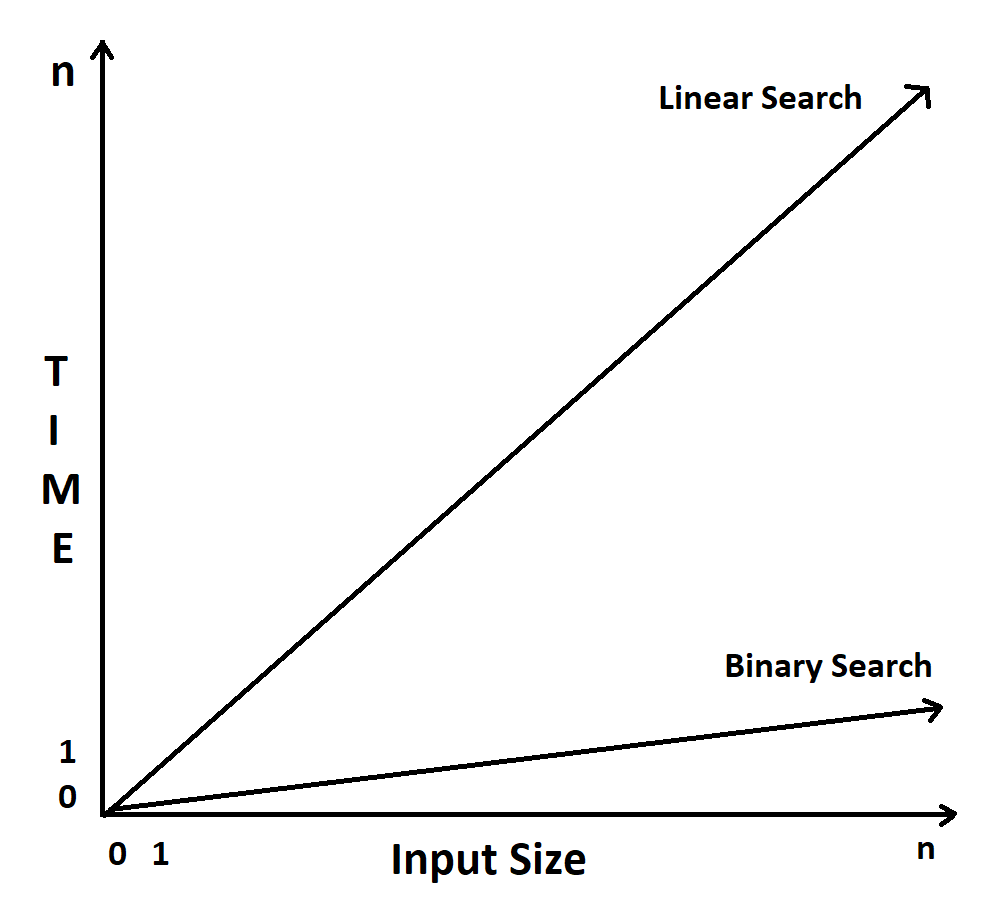In the above graph, we can see that the time taken by the linear search is directly proportional to the input size. Whereas the time taken by the Binary search is significantly less than the linear search.

### Applications of Binary Search

• Binary search can be applied to many problem-solving approaches, like finding a peak element in an array, searching an element in an array, finding the position of the element to be inserted on an array, etc.
• Deriving the concept of Binary Search, the concept is called Binary search tree. It is the tree data structure that uses the same approach as binary search and helps to find the node in the tree.

## Conclusion

Binary search is the search technique that helps to search the element faster. And this works only if the array is sorted. It uses the divide and search approach to search the element.